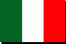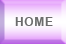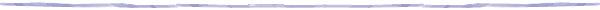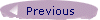The Calibration FilesThe calibration files are text file with the .rxg extension and are computer generated after the calibration operations.

For each receiver they contains mainly the FWHM, the gain curves and the calibration temperature as function of the frequency. The calibration temperature is given for fixed frequency values, in order to cover the whole receiver band. The calibration values for unlisted frequencies must be interpolated from the nearest values.

The following table shows how to read the data :

 Line Label Description 1 Receiver name 2 Fixed LO values (MHz) Range LO range of values (MHz) 3 Creation date (yyyy/mm/dd) 4 Constant FWHM (rad) Frequency FWHM constant (FWHM = 1.22 ∙ value ∙λ/D [rad]) 5 Available polarizations 6 Maximum gain (DPFU) for each polarization as listed above (K/Jy) 7 Elev Poly G(el) Normalized gain curve's polynomial coefficient (increasing powers) Altaz G(z) Normalized gain curve's polynomial coefficient (increasing powers) 8 and following Polarization, Frequency (MHz), Calibration temperature (K) end_tcal_table

D = diameter of the antenna

DPFU = Degrees Per Flux Unit

el = elevation

z = zenith distance

The KKC (22 GHz) file contains also the receiver noise temperature and the spillover temperature at various elevations :

 Noise temperature (K) Elevation (°), Spillover temperature (K) end_spillover_table